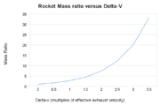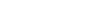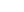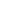xTsiolkovsky rocket equationOverview

The Tsiolkovsky rocket equation, or ideal rocket equation is an equation that is useful for considering vehicles that follow the basic principle of a rocket
Rocket
A rocket is a missile, spacecraft, aircraft or other vehicle which obtains thrust from a rocket engine. In all rockets, the exhaust is formed entirely from propellants carried within the rocket before use. Rocket engines work by action and reaction...

: where a device that can apply acceleration to itself (a thrust
Thrust
Thrust is a reaction force described quantitatively by Newton's second and third laws. When a system expels or accelerates mass in one direction the accelerated mass will cause a force of equal magnitude but opposite direction on that system....

) by expelling part of its mass with high speed and moving due to the conservation of momentum
Momentum
In classical mechanics, linear momentum or translational momentum is the product of the mass and velocity of an object...

. Specifically, it is a mathematical equation that relates the delta-v (the maximum change of speed of the rocket if no other external forces act) with the effective exhaust velocity and the initial and final mass of a rocket
Rocket
A rocket is a missile, spacecraft, aircraft or other vehicle which obtains thrust from a rocket engine. In all rockets, the exhaust is formed entirely from propellants carried within the rocket before use. Rocket engines work by action and reaction...

(or other reaction engine
Reaction engine
A reaction engine is an engine or motor which provides propulsion by expelling reaction mass, in accordance with Newton's third law of motion...

.)

For any such maneuver (or journey involving a number of such maneuvers):where:is the initial total mass, including propellant.is the final total mass.is the effective exhaust velocity.